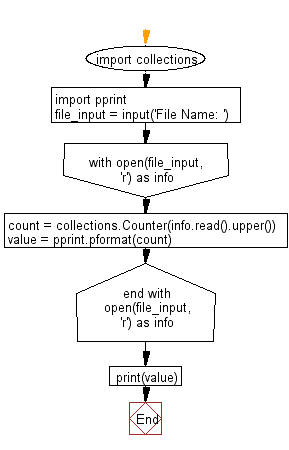﻿ Python: Count the number of each character of a given text of a text file - w3resource# Python: Count the number of each character of a text file

## Python Basic - 1: Exercise-7 with Solution

Write a Python program to count the number of each character of a text file.

Inputs:
abc.txt -
German Unity Day
The Day of German Unity (German: Tag der DeutschenEinheit) is the national day of Germany, celebrated on 3 October as a public holiday. It commemorates the anniversary of German reunification in 1990, when the goal of a united Germany that originated in the middle of the 19th century, was fulfilled again. Therefore, the name addresses neither the re-union nor the union, but the unity of Germany. The Day of German Unity on 3 October has been the German national holiday since 1990, when the reunification was formally completed.

Sample Solution:

Python Code :

``````import collections
import pprint
file_input = input('File Name: ')
with open(file_input, 'r') as info:
value = pprint.pformat(count)
print(value)
``````

Sample Output:

```File Name:  abc.txt
Counter({' ': 93,
'E': 64,
'N': 45,
'A': 42,
'T': 40,
'I': 36,
'O': 31,
'R': 29,
'H': 25,
'D': 19,
'M': 17,
'Y': 17,
'L': 15,
'F': 15,
'U': 14,
'C': 13,
'G': 13,
'S': 12,
',': 7,
'B': 6,
'W': 5,
'9': 5,
'.': 4,
'P': 4,
'1': 3,
'\n': 2,
'0': 2,
'3': 2,
':': 1,
'-': 1,
'K': 1,
'(': 1,
')': 1,
'V': 1})
```

Flowchart:Python Code Editor :

Have another way to solve this solution? Contribute your code (and comments) through Disqus.

What is the difficulty level of this exercise?

Test your Python skills with w3resource's quiz

﻿

## Python: Tips of the Day

Checks if the given number falls within the given range.

Example:

```def tips_range(n, start, end = 0):
return start <= n <= end if end >= start else end <= n <= start
print(tips_range(2, 4, 6))
print(tips_range(4, 8))
print(tips_range(1, 3, 5))
print(tips_range(1, 3))
```

Output:

```False
True
False
True
```